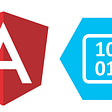# Quick Big O Intro

Have you ever hear the phrase “There are a million different ways to skin a cat”, and wondered which one would be the best way? That is sort of like the concept of Big O when it comes to software engineering.

Say you write two codes that can do the same function. Which one do you choose to use? Why did you choose this one? When we are thinking about Big O, we are looking to see what is the best code to use, and how the runtime of an algorithm grows as the inputs grow. We typically want to look at the time it takes for the code to be completed, and how much additional memory we need to allocate in order to run a code in our algorithm. This is Time Complexity and Space Complexity.

# Time Complexity:

When looking to see the time complexity with a function, we want to look at how long it takes code to execute. There is however a problem with time because different machines will record different times, and the same machine could also record different times. There is also a chance that when dealing with a fast algorithm, speed measurements may not be precise enough.

With that said, rather than count the numbers of seconds a code takes to execute, we want to count the number of simple operations the computer has to perform because that is always constant.

Simple Operator Examples: Multiply, Divide, Add, Subtract, Assignments, Comparisons

There are typically 3 types:

• Constant: When there will always be the same amount of operators no matter what. O(f(n)=(1)).
• Linear: When the number of operations grow roughly in proportionate with (n). O(f(n)=(n)).
• Quadratic: Typically exists when you are dealing with nested loops. As (n) grows, the runtime roughly grows at the rate of n². O(f(n)=(n²)).

# Space Complexity:

We can use the same formal syntax we have been using for time complexity with space. However it is important to note that when we are discussing space complexity, we are really talking about auxiliary space complexity because we only want to know how much space is required by the algorithm, not including the space taken up by the inputs.

Below are some basic rules of thumb when dealing with space complexity:

• Most primitives (booleans, numbers, undefined, null) are constant space.
• Strings require O(n) (space where n is the string length)
• Reference types, arrays, and objects are generally O(n), where n is the length(for arrays) or the number of keys (for objects)

And this a just a brief rundown discussing the concept of Big O.

--

--

--

## More from Thomas Muscarello

Software Engineer

Love podcasts or audiobooks? Learn on the go with our new app.

## Node.js 8: Big Improvements for the Debugging and Native Module Ecosystem## 2022 Tech Stack, Next.js## Upload to Azure Blob Storage with Angular## How to remove falsy values from an array in JavaScript## Load Balancer with Node & Nginx## How to send emails securely using Gmail and NodeJS## To Infinity and JSON!## Thomas Muscarello

Software Engineer

## Leetcode 238: Product of Array Except Self Javascript solution## Using a JavaScript Hash Table To Lower Big O Time Complexity When Searching For The First Recurring…## Phase-2 “REACT.JS”## What is Depth-First Search?